# Boxes to measure and carry objects

Many objects come with a box around them which is useful to measure and carry. The simplest way to move objects is to indicate, when they have a box, how to put the box relatively to an other box. For instance, to put a cube above a cylinder, proceed as follows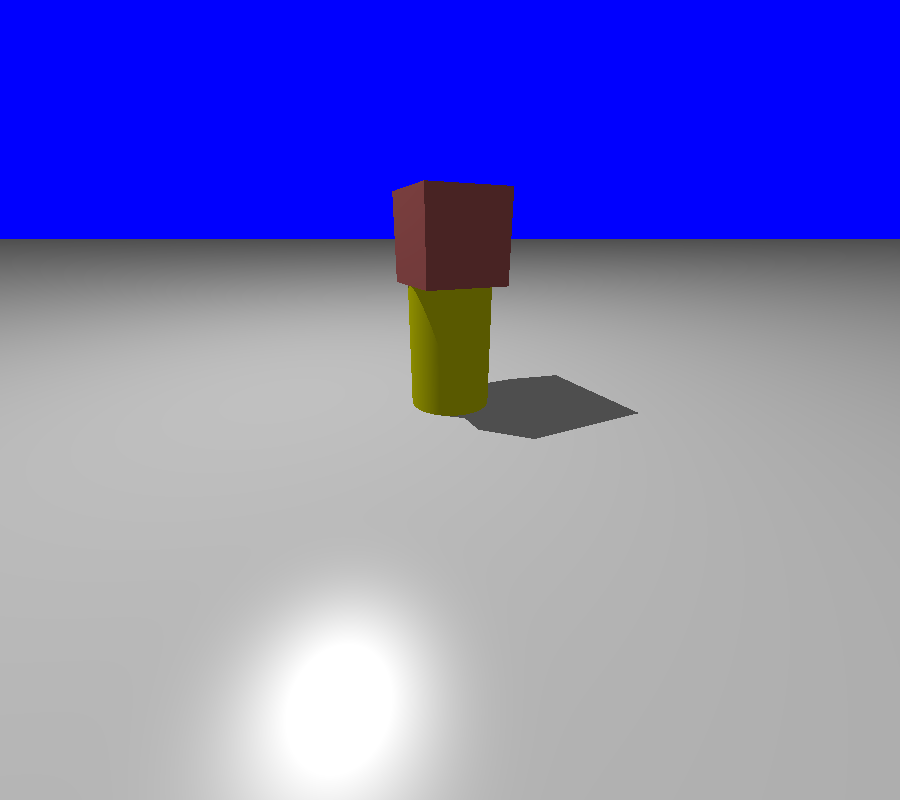g=plane(Z,origin).colored("Grey")
cyl=Cylinder(origin,origin+1.5*Z,.5).colored("Yellow")
myCube=Cube(1,1,1).colored("Brown").above(cyl)

camera.filmAllActors=False
camera.file="cubeOverCyl.pov"
camera.location=origin-4.3*Y+2.*Z-2*X
camera.zoom(.15)
camera.lookAt=origin
camera.actors=[cyl,myCube,g]
camera.shoot
camera.show


Similarly, there are commands object1.below(object2), object1.on_left_of(object2), object1.on_right_of(object2), object1.in_front_of(object2), object1.behind(object2).

But quite often, these commands are not sufficient. First, one sometimes need to make a rotation to the box before translating it. Second, when there are two boxes of different sizes, it is possible to adjust the centers, or the edges, or the corners...

We explain below the general formalism of boxes.

## Visualize the current box

The boxes are useful for the computations but they are not visible by default. We may visualize them when we do not remember the size or the orientation of the box.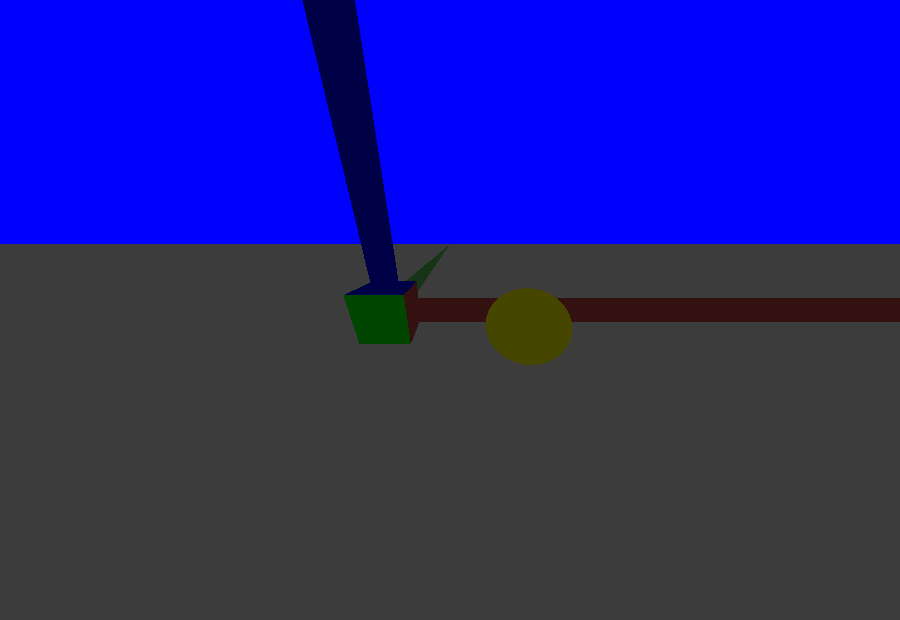As an example, we draw two spheres s,t, and we show the box of the small sphere s.

Note that the colors of the facets correspond to the local axis of the object (XYZ corresponds to the colors RGB). A half cylinder indicates the positive direction on each coordinate. For instance, for the small sphere in the box, the local Y coordinates is in front of us, along the green cylinder. (Not seen on this picture: The side facing the negative coordinates is lighter than the facet which faces the positive coordinates)

g=plane(Z,origin-Z)
g.color="Grey"
s=Sphere(origin-2*X,.79)
t=Sphere(origin+2*X-Y,1)
t.color="Yellow"

s.show_box()

camera.filmAllActors=False
camera.file="show_box.pov"
camera.location=origin-4.3*Y+2.*Z
camera.zoom(.65)
camera.lookAt=origin
camera.actors=[s,t,g]
camera.shoot
camera.show


## Measurement relative to a box.

When the object is not a cube, it is convenient to imagine that the object is wrapped by a carton box, and we may take measurements relative to the carton box wrapping the object.

When we make a measurement of a piece of wood on the desk, sometimes we start from the left side (“5 centimeters from the left side of the desk”) sometimes from the right side (“5 centimeters from the right side”) sometimes we describe the points in terms of proportionality (“in the middle between the left side and the right side”). These three possibility of measurements are possible inside a box with pycao. They correspond to the frames “a” (for absolute), “n” (for negative) and “p” (for proportional). Also you can mix the units of measurements on each axis. For instance, if you choose “anp”, ie absolute for x, negative for y and proportional for z, a point with coordinate [x,y,z]=[.5,.5,.5] will be inside the box .5 units from the face with minimal x, .5 units from the face with maximal y, and in the middle of the the two planes with constant z.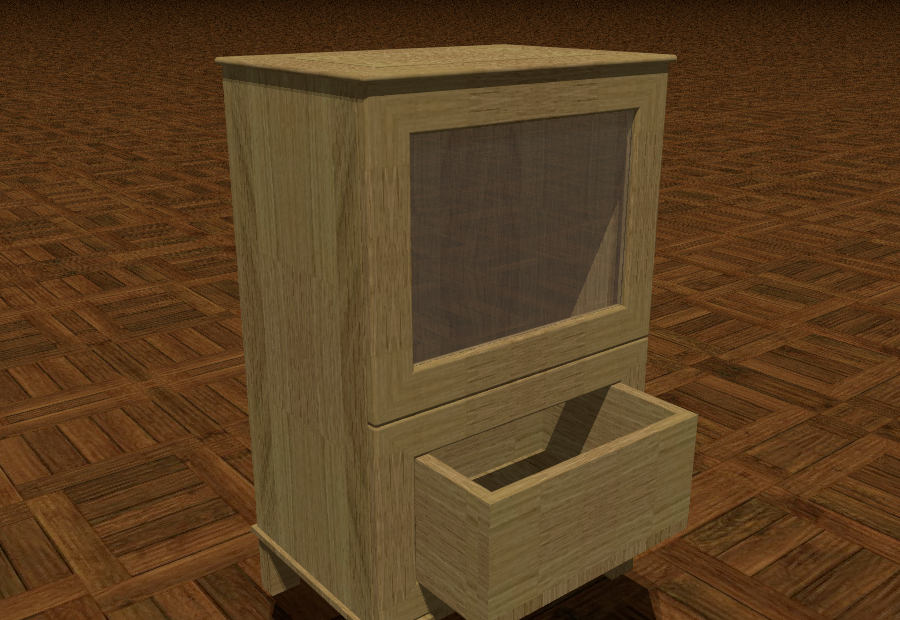To illustrate these notations, we draw a little drawer. The code uses instructions “object.point(x,y,z,”rst”)” which define a point relative to the bounding box of the object, where the letters “rst” have value “a”,”n” or “p”.

pass
g=plane(Z,origin-Z).colored("Grey")
#g.color="Grey"
boardThickness=.02
drawer=Cube(.4,.4,.1).colored("Brown")
# We define two points which are the opposite corners of the cube toCut
firstPoint=drawer.point(boardThickness,boardThickness,boardThickness,"aaa")
secondPoint=drawer.point(boardThickness,boardThickness,1.1,"nnp")
toCut=Cube(firstPoint,secondPoint).colored("Yellow")
drawer.amputed_by(toCut)
button=Sphere(drawer.point(.5,0,.5,"pap"),.01).colored("Yellow").glued_on(drawer)

camera=Camera()
camera.filmAllActors=False
camera.location=origin-3.3*Y+2.*Z
camera.file="drawer.pov"
camera.zoom(1.5)
camera.povraylights="light_source {<-2,0,6.8> color White " + "}\n\n"
camera.lookAt=drawer.center
camera.actors=[g,drawer]
camera.shoot
camera.show


## Lines and planes in a box

We have seen the notations to describe a point relative to a box. Similarly, planes parallel to the facets and lines perpendicular to the facets of the box of a bounded objects are easily accessible to be used as intermediate steps of the construction.

Here is an example, where we draw infinite cylinders along the lines and an orange plane.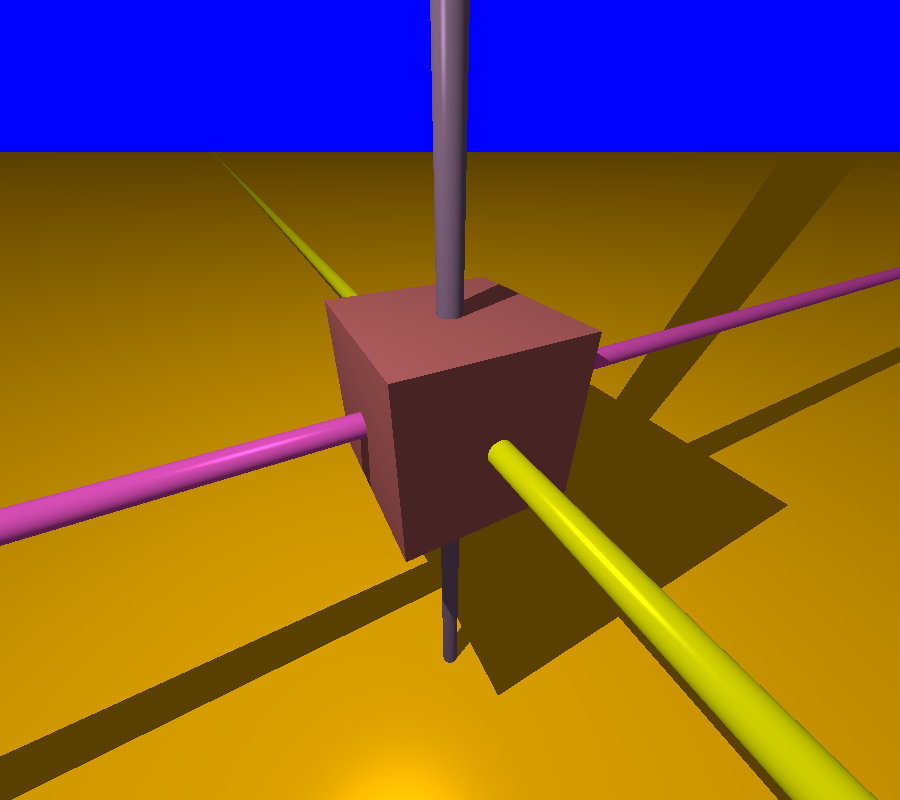As for the code, the lines are described by an instruction object.segment(x,y,z,”rs”). The coordinate that moves along the line is described as “None” and r,s have value a,n or p according to the frame.Such a plane is described by an instruction object.plane(A,”r”), with A=+/- X,Y or Z.

w=Cube(3,3,3).colored('Brown')
seg=w.segment(None,0.5,0.5,"ppp") # a line where xloc,yloc are fixed and zloc varies
cyl=ICylinder(seg,0.2).colored('SpicyPink')
seg2=w.segment(0.5,None,0.5,"ppp")
cyl2=ICylinder(seg2,0.2).colored('Yellow')
seg3=w.segment(0.5,0.5,None,"ppp")
cyl3=ICylinder(seg3,0.2).colored('Violet')
p=w.plane(Z,-2.5,"p")
p.color="Orange"

camera=Camera()
camera.filmAllActors=False
camera.file="lineInABox.pov"
camera.location=origin-X-3.3*Y+5.*Z
camera.zoom(.15)
camera.povraylights="light_source {<-2,0,6.8> color White " + "}\n\n"
camera.lookAt=w.center
camera.actors=[w,cyl,cyl2,cyl3,p]
camera.shoot
camera.show


## Carrying object with boxes.

When someones asks to put a sofa parallel to a wall, the sofa is assimilated to a cube and what is asked is to put one edge parallel to the walll. In Pycao, we mimic the natural language : We assimilate an object to its box, or equivalently we move the box and we carry the object in the box.

As an example, we consider the following scene.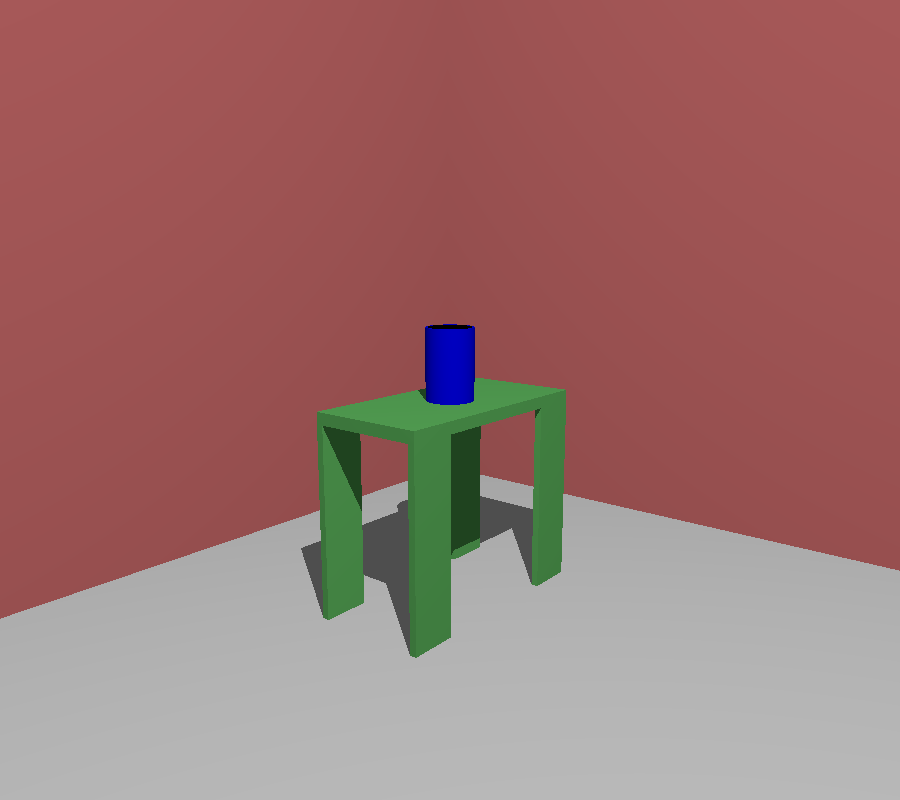# The dimensions/constants used are in the next 6 lines
################
tableTrayDimensions=Vector(1,.5,.05)
tableLegDimensions=Vector(.2,.03,.8)
placementVector=X+Y
flowerPotHeight=0.3
flowerPotThickness=0.01

# Describing the scene starts here
################
ground=plane(-Z,origin).colored("Grey") # a plane with normal vector Z=(0,0,1) through the origin
wall1=plane(X,origin).colored("Brown")
wall2=plane(Y,origin).colored("Brown")
tableTray=Cube(tableTrayDimensions).colored("ForestGreen")
tableLeg1=Cube(tableLegDimensions).colored("ForestGreen")
tableLeg2=tableLeg1.copy()
tableLeg3=tableLeg1.copy()
tableLeg4=tableLeg1.copy()

# The next 2 lines correspond to the movement of tableLeg1 to the
# corner of the tray  and to the bonding of the leg on the tray
#################

# The tray is moved and the legs follow because of the bonding
################
bottomOfTheLeg1=tableLeg1.point(0,0,0,"aap")
tableTray.translate(origin-bottomOfTheLeg1) # vertical move: legs on the floor
tableTray.translate(placementVector) # horizontal move

# The flower pot is described as a difference between 2 cylinders then
# placed on the table
################
flowerPot.amputed_by(toCut)
topCenterOfTable=tableTray.point(0.5,0.5,1,"ppp")
flowerPot.translate(topCenterOfTable-origin)

camera=Camera()
camera.filmAllActors=False
camera.location=origin+4*X+3.3*Y+1.5*Z
camera.file="table.pov"
camera.zoom(.315)
camera.povraylights="light_source {<+4,4,4.8> color White " + "}\n\n"
camera.lookAt=topCenterOfTable
camera.actors=[ground,wall1,wall2,tableTray]
camera.shoot
camera.show


## Faces of a box.

The above code requires some notation to be explained.

Starting from its center, an object admits 6 faces of dimension 2 denoted by X,-X,Y,-Y,Z,-Z which correspond to the colored facets in the above example. Remember that this refers to the local coordinate of the object, they are not not parallel to the global frame of the 3D space.

Similarly, there are 12 edges, denoted by X+Y,X-Y,.... Y-Z.

And there are 8 corners denoted by X+Y+Z,....-X-Y-Z.

## Moving a box against an other box.

When we move a box A against a box B, we must do two things: orientate the box A so that the faces of A are parallel to the faces of B, then we must choose the faces of contact ( putting A above or below B, on the left or on the right...)

The command

A.against(B,faceA1,faceB1,faceA2,faceB2)


means that:

• the orientation of A is such that :
• faceA1 and faceB1 are positivly parallel
• faceA2 and faceB2 are positivly parallel
• FaceA1 is the face of contact of A between A and B.
• The faces of A and B in contact have the same center

For instance, in the following code, we take conventions of colors RGB for the axis XYZ. The instruction yellowCube.against(brownCube,-Z,Y,X,X) means that vectors -Z (opposite to the blue half-line) of the yellow cube is in the same direction that the Y ( green) of the Brown Cube. The face -Z of YellowCube is indeed a face of contact. And the two X axis (Red) are positivly parallel.Suppose that we want to move the yellow cube to the left till the edges of both Cubes coincide. We need to push Yellow Cube in direction opposite to the red, ie. -X. Thus we add the option ajustedges=-X ie we put yellowCube.against(brownCube,-Z,Y,X,X,adjustEdges=-X)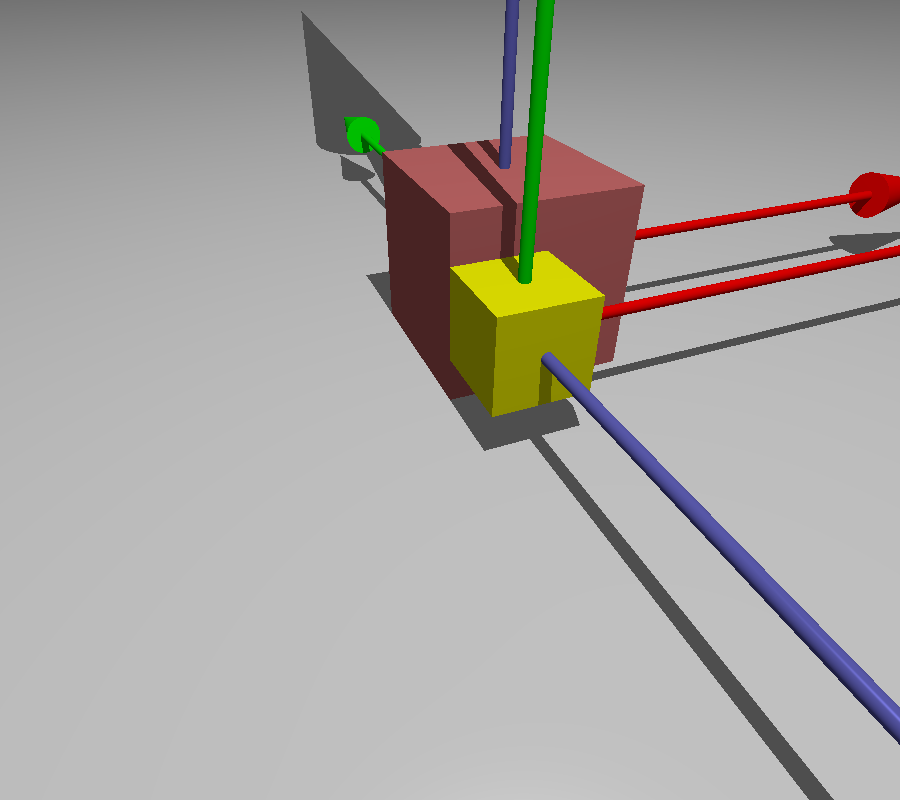With yellowCube.against(brownCube,-Z,Y,X,X,adjustEdges=X+Y), the yellow Cube goes to the upper right. With, yellowCube.against(brownCube,-Z,Y,X,X,adjustEdges=0.8*(X+Y), it nearly goes to the upper right, as shown-below.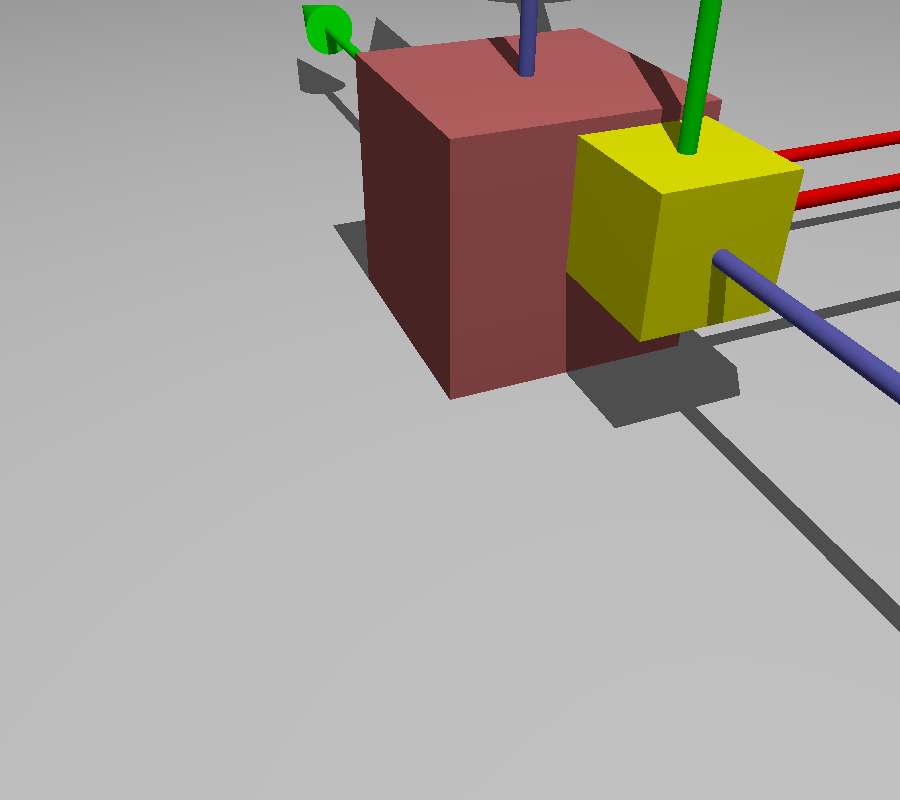If we want to put the yellow cube off the brown cube, we can add an offset. The offset is in global coordinates. In our picture, the yellow cube has been rotated, but the brown cube has not moved so its coordinates are still parallel to the global axis of the scene. Thus the offset must be a negative mutltiple of the green axis Y of the Brown Cube. For instance, we may put yellowCube.against(brownCube,-Z,Y,X,X,adjustEdges=.8*(X+Y),offset=-0.7*Y)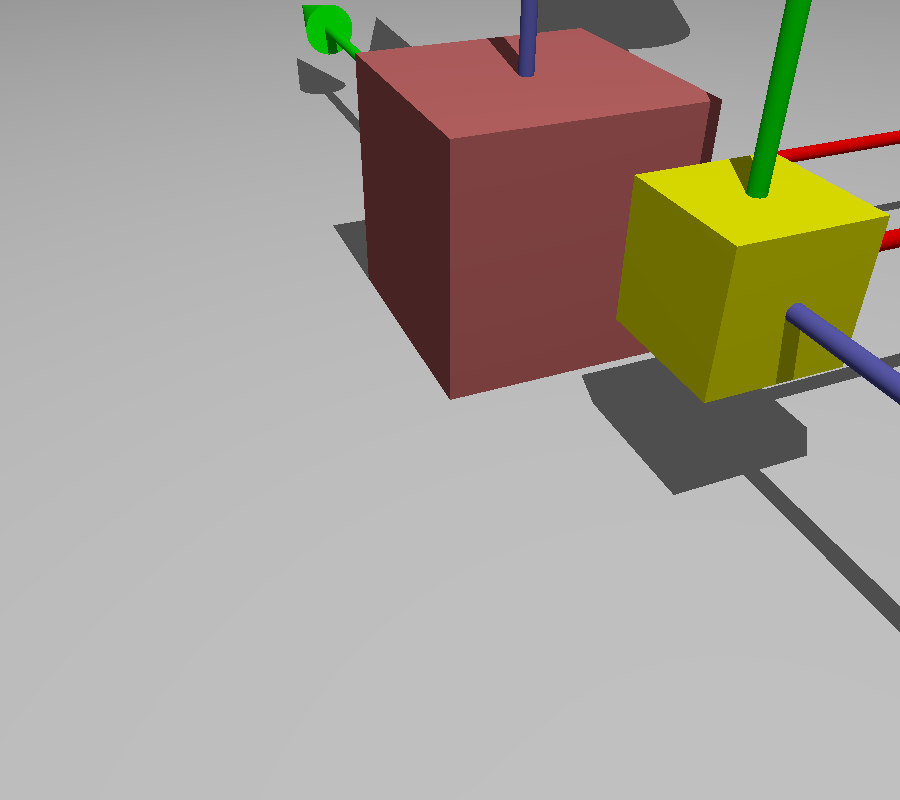Finally, it is possible to add the option adjustAxis=[p1,p2]. The adjustement computed by Pycao is a move parallel to the face of contact (in our case, the -Z face of YellowCube) so that p1 and p2 are on a line parallel to the blue axis of YellowCube. To illustrate, this notion we make the corners of YellowCube and BrownCube coincide.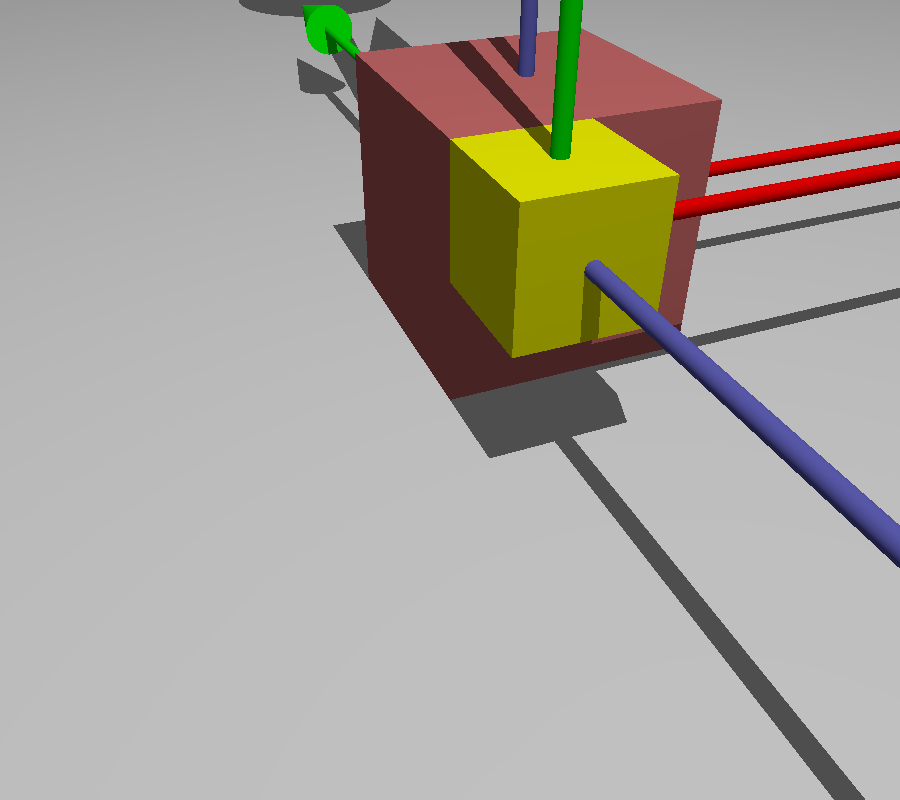axisLength=0.8
axisThickness=0.06
arrowThickness=.3
brownCube=Cube(2,2,2) .colored("Brown")# the cube is moved above the plane
ground=brownCube.plane(Z,0,"a") .colored('Gray' )
yellowCube=Cube(1,1,1).colored("Yellow") # the cube is moved above the plane

xaxis=FrameAxis(brownCube.center,brownCube.center+7*X,axisLength,axisThickness,arrowThickness).colored('Red').glued_on(brownCube)
yaxis=FrameAxis(brownCube.center,brownCube.center+7*Y,axisLength,axisThickness,arrowThickness).colored('Green').glued_on(brownCube)
zaxis=FrameAxis(brownCube.center,brownCube.center+7*Z,axisLength,axisThickness,arrowThickness).colored('NavyBlue').glued_on(brownCube)

xaxisg=FrameAxis(yellowCube.center,yellowCube.center+10*X,axisLength,axisThickness,arrowThickness).colored('Red').glued_on(yellowCube)
yaxisg=FrameAxis(yellowCube.center,yellowCube.center+10*Y,axisLength,axisThickness,arrowThickness).colored('Green').glued_on(yellowCube)
zaxisg=FrameAxis(yellowCube.center,yellowCube.center+10*Z,axisLength,axisThickness,arrowThickness).colored('NavyBlue').glued_on(yellowCube)

upperLeftBrownPoint=brownCube.point(0,0,1,"ppp")
upperLeftYellowPoint=yellowCube.point(0,1,1,"ppp")

camera=Camera()
camera.location=origin-5*Y+4*Z-2*X
camera.filmAllActors=False
camera.actors=[ground,brownCube,yellowCube,xaxis,yaxis,zaxis,xaxisg,yaxisg,zaxisg] # what is seen by the camera   camera.lookAt=.5*(basket2+basket)
camera.zoom(.3512)
camera.shoot # takes the photo, ie. creates the povray file, and stores it in camera.file
camera.show # show the photo, ie calls povray.


## Relative placement: Above,behind,on_left_of...

Left, right, up are relative notions. The instruction object1.above(object2,adjustEdges,offset) is a synononym of object1.against(object2,-Z,-Z,X,X,adjustEdges,offset)

To say the things differently, the instructs, above, behind, in_front_of .... suppose that we see the object from a point (0,-y,0) with y large enough, that the frame that we use is direct and that the gravity vector is (0,0,-g) in this frame.

## Adding new boxes

It may happen that we want to create a box on an object which does not have one. For instance the ruled surfaces do not have any box with them at creation time. Or we want to use several boxes on a complex object. Here is an example of code to add boxes, and select one. The selected box is the box use in the against function.

firstBox=FrameBox([origin,point(2,5,7), point(1,1,1)]) # The smallest box containing the points in the list
secondBox=FrameBox([origin, point(-1,1,1)]) #  An other box
myObject=point(0,0,0)
myObject.add_box("newbox",firstBox) # adds the box to the point and select it
print(myObject.box())
print (myObject.box()) # returns the information on the second Box
myObject.print_boxes() #  the names of the boxes of a
myObject.select_box("newbox") # selects firstBox again
print (myObject.box())


The output is:

FrameBox:
Origin: Affine Point  [0.0,0.0,0.0]
Vectors:
Vector  [2.0,0.0,0.0]
Vector  [0.0,5.0,0.0]
Vector  [0.0,0.0,7.0]

FrameBox:
Origin: Affine Point  [-1.0,0.0,0.0]
Vectors:
Vector  [1.0,0.0,0.0]
Vector  [0.0,1.0,0.0]
Vector  [0.0,0.0,1.0]

['mySecondBox', 'newbox']
FrameBox:
Origin: Affine Point  [0.0,0.0,0.0]
Vectors:
Vector  [2.0,0.0,0.0]
Vector  [0.0,5.0,0.0]
Vector  [0.0,0.0,7.0]